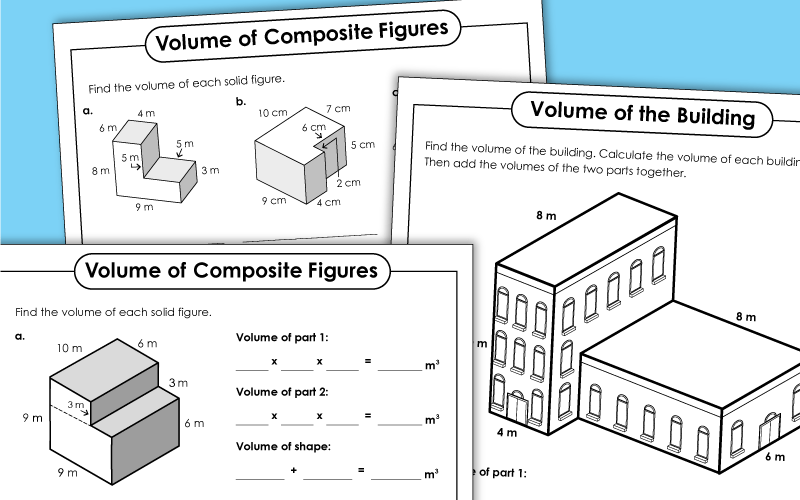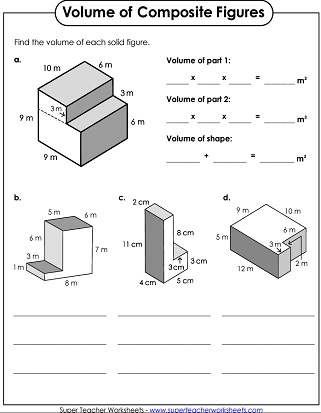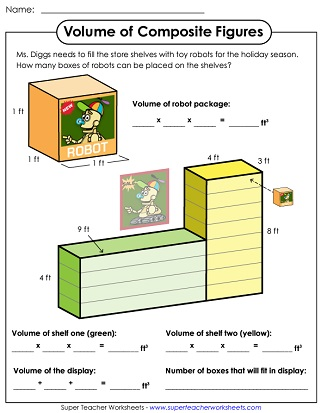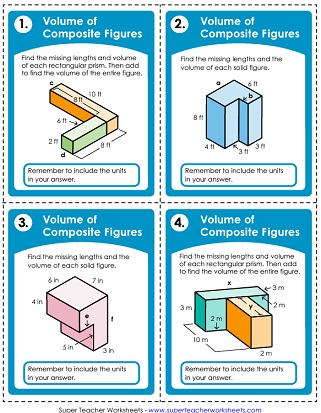# Volume of Composite Figures (Additive Volume)

On these worksheets, students calculate the volumes of composite figures. To do this, they find the volumes of the two (or more) rectangular prisms that make up the shape; then they add them together. These activities align with Common Core Standard 5.MD.5c.## Basic: All Lengths GivenSingle, Whole Number Lengths

Find the additive volume of four composite figures. The first one is a step-by-step example. This worksheet has 4 problems to solve.
This worksheet has two composite shapes for students to practice volume calculations with.
Find the volume of each composite shape, made up of rectangular prisms. On this worksheet, the lengths of all sides are given.
Use this worksheet to show students how to solve a real-life composite volume problem.
Use these task cards with students learning the basics of composite-figure volume.
The building show in made up of two rectangular prisms. Find the volume of each part and add them together.
Students can use this worksheet to find the volume in pixels of a video game character.
With this basic-level worksheet, students find the volumes of two different RVs and determine which is larger.
Figure out how many boxes of robots can fit on the shelves using concepts of composite volume.

## Intermediate: Some Missing LengthsSingle, Whole Number Lengths

Use additive calculations to find the volumes of four figures. Each figure is made of non-overlapping rectangular prisms. Two side lengths not given for each figure.
This worksheet has two figures for students to find the missing lengths and volumes of.
This worksheet has six additive volume problems to solve. Not all sides are given.
This word problem helps students understand when they may need to find composite volume in the real world.
These task cards can help students practice finding the volume of composite figures.
Students must find the lengths of three sides of this composite figure to be able to find the volume of the video game character.
With this worksheet, students must find the lengths of two sides of two different composite figures and then calculate each shape's volume in order to determine which is bigger.
This worksheet is slightly more difficult than the basic Stocking the Shelves worksheet.

## Advanced: Some Missing LengthsDecimal & 2-Digit Lengths

Find the volume of each figure. Sides are either 2-digit lengths or decimals. Not all sides are given. First problem is a walk-through style.
This worksheet includes two composite figures for students to find the missing lengths and volumes of.
Students can use concepts of additive volume to find the volumes of these six shapes.
Use these task cards to help reinforce key skills related to finding the volume of composite figures.
To find the volume of this video game character, students must first determine the lengths of three sides of the composite figure.
Practice finding composite volume with this advanced worksheet featuring two diagrams of RVs.
Find the missing lengths and calculate the volume of each shelf to find out how many robot boxes can fit on all the shelves.
Volume of Rectangular Prisms (Basic)

Multiply length × width × height to find the volumes of the rectangular prisms.

More Volume Worksheets

This page will connect you to collections of worksheets for teaching basic volume of rectangular prisms, as well as volume of spheres, cones, and cylinders.

Area Worksheets

Calculate the areas of rectangles, triangles, parallelograms, trapezoids, and irregular shapes. Also includes surface area worksheets.

## Sample Worksheet ImagesMy Account
Site Information